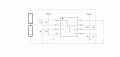# Building a Differential Amplifier PROBLEM need helps !!!

#### Ian0

Joined Aug 7, 2020
3,277
What output voltage do you get?
What is the power supply voltage for your LM358?

#### Tài Dương

Joined Jun 15, 2017
10
What output voltage do you get?
What is the power supply voltage for your LM358?
output voltage i get with v2=8v, v1=4v is 4,2v and 4,4v for 2 output in lm358
supply voltage is 8v from the battery

#### KeepItSimpleStupid

Joined Mar 4, 2014
5,090
8-=4 is ~4, Which, in theory is correct if ideal. The real OP amp isn't ideal.

What non-ideal issues are causing problems?

•Tài Dương

#### Ian0

Joined Aug 7, 2020
3,277
8-=4 is ~4, Which, in theory is correct if ideal. The real OP amp isn't ideal.

What non-ideal issues are causing problems?
That's a LOT of offset. 5mV would be the maximum for an LM358 unless the gain resistors very large

There are obviously two circuits here - how are they connected?
What are the real voltages on the battery? I would doubt that they are both 4.000V

#### Tài Dương

Joined Jun 15, 2017
10
8-=4 is ~4, Which, in theory is correct if ideal. The real OP amp isn't ideal.

What non-ideal issues are causing problems?
i need to get accurate battery voltage to balance the battery pack

#### Tài Dương

Joined Jun 15, 2017
10
That's a LOT of offset. 5mV would be the maximum for an LM358 unless the gain resistors very large

There are obviously two circuits here - how are they connected?
What are the real voltages on the battery? I would doubt that they are both 4.000Vi wired like this picture
bat 1 = 3.64v
bat 2 = 3.61v

Vout 1 = 3.62v
Vout 2 = 3.68v
this is excalty voltage in my circuit

#### Ian0

Joined Aug 7, 2020
3,277
So both circuits measure the output of the top battery, and neither measures the output of the lower one?
Those offsets are rather more realistic and about what you could expect for a LM358 and 1% resistors.
If you need better accuracy (why?) then use an OP07 or TLV07 and 0.1% resistors.

•Tài Dương